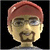## Convert fraction to decimal numbersTags:
Conversion functions
Visual Basic
In Visual Basic, how do you convert fraction to decimal numbers?

Thanks. We'll let you know when a new response is added.

I’m not sure what you mean; afaik, VB doesn’t have a Fraction type. But in general, you can get a decimal number by dividing the numerator by the denominator.

If you have a string that represents a fraction, e.g. “4/9”, you can split the string on the “/” character. You can then use CInt(str) to convert each string-as-number to an Integer (or CLng if you need a long), but make sure to call IsNumeric(str) on each string-as-number before you try to convert it, because CInt/CLng do not error out quietly.

If you have a string that contains one of the fractional special characters, e.g. “¾”, I think you’ll have to just manually do the conversion with a select statement or equivalent.

```Public Function GetNumerator(ByVal x As Decimal, ByVal denominator As Integer) As Decimal
x = x Mod 1
'get numerator
x = x * denominator
'round to whole number
Return Decimal.Round(x, 0)
End Function

Public Function GetGCF(ByVal x As Integer, ByVal x1 As Integer) As Integer
x = Math.Abs(x)
x1 = Math.Abs(x1)
If x = x1 Then Return x
If x > x1 Then
If x Mod x1 = 0 Then
Return x1
Else
For i As Integer = x1 - 1 To 1 Step -1
If x Mod i = 0 And x1 Mod i = 0 Then
Return i
End If
Next
End If
Else
If x1 Mod x = 0 Then
Return x
Else
For i As Integer = x - 1 To 1 Step -1
If x1 Mod i = 0 And x Mod i = 0 Then
Return i
End If
Next
End If
End If
End Function

Public Function GetRoundedFraction(ByVal d As Decimal, ByVal Maxd As Integer) As String
Dim Numerator As Integer = GetNumerator(d, Maxd)
Dim GCF As Integer = GetGCF(Numerator, Maxd)

If GCF = 0 Then Return "0"
Numerator = CInt(Numerator / GCF)
Dim Denominator As Integer = CInt(Maxd / GCF)
Return (Numerator & "/" & Denominator)
End Function```

## Discuss This Question: 3 Replies

Thanks. We'll let you know when a new response is added.
• Hsmithdp, doesn't that do the opposite? That is, doesn't it take a decimal and turn it into a fraction? Also, in VBA (at least the one I have, ymmv) you can't declare a variable to be of type Decimal -- probably want a Double instead. And similarly, you can't return a value like that -- you have to set the function to the value, and then exit the function. So instead of:
```Public Function getNumberThreeOrFour(makeItThree as Boolean) as Integer
If makeItThree Then
return 3
Else
return 4
End If
End Function```
You would have:
```Public Function getNumberThreeOrFour(makeItThree as Boolean) as Integer
If makeItThree Then
getNumberThreeOrFour = 3
Exit Function
Else
getNumberThreeOrFour = 4
Exit Function
End If
End Function```
(Of course, that's a silly function and even that could be done better with a single line using IIF statement, but it's just to illustrate the point.)
report
•Possibly I'm missing something. YuvalShavit seems to be on the right track here. But it seems to be a simple math question. The fraction 2/3 is a division problem (2 divided into 3), so a double will hold that type of number. In vbscript it would be: Function GetDecimal(numerator, denominator) GetDecimal = numerator / denominator End Function In VB it would be as simple as: Public Function GetDecimal(numerator as integer, denominator as integer) as double GetDecimal = numerator / denominator End Function In a VB class it would be: Public Shared Function GetDecimal(numerator as integer, denominator as integer) as double GetDecimal = numerator / denominator End Function As far the difference in the variable types I recently wrote about this in my blog here on ITKE. Check it out under variable types.
report
• The GetDecimal function is fine, but what to do with division by zero? Because the return type is double, the function will return the "correct" result (Infinity) but an overflow error will occur if any attempt is made to use rather than just report the result. Choices? 1) Check for zero denominator and throw an exception 2) Check for return value as in:
```        Dim DEC As Double
DEC = GetDecimal(0, 0)
If (DEC.ToString = "Infinity") Or (DEC.ToString = "NaN") Then
System.Console.WriteLine("Divide by zero error")
End If```
report